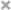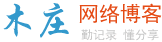# 解读C#中的正则表达式

regexp规则类包含在System.Text.RegularExpressions.dll文件中，在对应用软件进行编译时你必须引用这个文件：

System.Text.RegularExpressions.dll

Capture: 包含一次匹配的结果；

CaptureCollection: Capture的序列；

Group: 一次组记录的结果，由Capture继承而来；

Match: 一次表达式的匹配结果，由Group继承而来；

MatchCollection: Match的一个序列；

MatchEvaluator: 执行替换操作时使用的代理；

Regex: 编译后的表达式的实例。

Regex类中还包含一些静态的方法

Escape: 对字符串中的regex中的转义符进行转义；

IsMatch: 如果表达式在字符串中匹配，该方法返回一个布尔值；

Match: 返回Match的实例；

Matches: 返回一系列的Match的方法；

Replace: 用替换字符串替换匹配的表达式；

Split: 返回一系列由表达式决定的字符串；

Unescape:不对字符串中的转义字符转义。

``Match m = Regex.Match("abracadabra", "(a|b|r)+"); ``

``if (m.Success)...``

``Console.WriteLine("Match="+m.ToString()); ``

``string s = Regex.Replace("abracadabra", "abra", "zzzz"); ``

``string s = Regex.Replace(" abra ", @"^\s*(.*?)\s*\$", "\$1"); ``

C#中，我们还经常使用字母字符串，在一个字母字符串中，编译程序不把字符“ \” 作为转义字符处理。在使用字符“\”指定转义字符时，@"..."是非常有用的。

``````string text = "abracadabra1abracadabra2abracadabra3";
string pat = @"
( # 第一个组的开始
abra # 匹配字符串abra
( # 第二个组的开始
)? # 第二个组结束（可选）
) # 第一个组结束
+ # 匹配一次或多次
";

//利用x修饰符忽略注释
Regex r = new Regex(pat, "x");

//获得组号码的清单
int[] gnums = r.GetGroupNumbers();

//首次匹配
Match m = r.Match(text);

while (m.Success)
{
//从组1开始
for (int i = 1; i < gnums.Length; i++)

{

Group g = m.Group(gnums[i]);

//获得这次匹配的组
Console.WriteLine("Group"+gnums[i]+"=["+g.ToString()+"]");

//计算这个组的起始位置和长度
CaptureCollection cc = g.Captures;

for (int j = 0; j < cc.Count; j++)

{

Capture c = cc[j];

Console.WriteLine(" Capture" + j + "=["+c.ToString()

+ "] Index=" + c.Index + " Length=" + c.Length);

}
}
//下一个匹配
m = m.NextMatch();
}
``````

``````Group1=[abra]

Capture1=[abra] Index=7 Length=4

Group1=[abra]

Capture1=[abra] Index=19 Length=4

Group1=[abra]

Capture1=[abra] Index=31 Length=4

``````

``````string text = "the quick red fox jumped over the lazy brown dog.";
System.Console.WriteLine("text=[" + text + "]");
string result = "";
string pattern = @"\w+|\W+";
foreach (Match m in Regex.Matches(text, pattern))
{

// 取得匹配的字符串
string x = m.ToString();

// 如果第一个字符是小写
if (char.IsLower(x))

// 变成大写
x = char.ToUpper(x) + x.Substring(1, x.Length-1);

// 收集所有的字符
result += x;
}

System.Console.WriteLine("result=[" + result + "]");``````

``````text=[the quick red fox jumped over the lazy brown dog.]
result=[The Quick Red Fox Jumped Over The Lazy Brown Dog.]
``````

``````static string CapText(Match m)

{

//取得匹配的字符串

string x = m.ToString();

// 如果第一个字符是小写

if (char.IsLower(x))

// 转换为大写

return char.ToUpper(x) + x.Substring(1, x.Length-1);

return x;

}

static void Main()

{

string text = "the quick red fox jumped over the

lazy brown dog.";

System.Console.WriteLine("text=[" + text + "]");

string pattern = @"\w+";

string result = Regex.Replace(text, pattern,

new MatchEvaluator(Test.CapText));

System.Console.WriteLine("result=[" + result + "]");

} ``````

c#之正则表达式介绍

c#中正则表达式有什么作用？匹配字符有什么含义？

c#中常用的正则表达式总结分享

asp.net 验证正则表达式方法总结

c#正则表达式元字符详解### 评论

• 欢迎访问木庄网络博客
• 可复制：代码框内的文字。
• 方法：Ctrl+C。